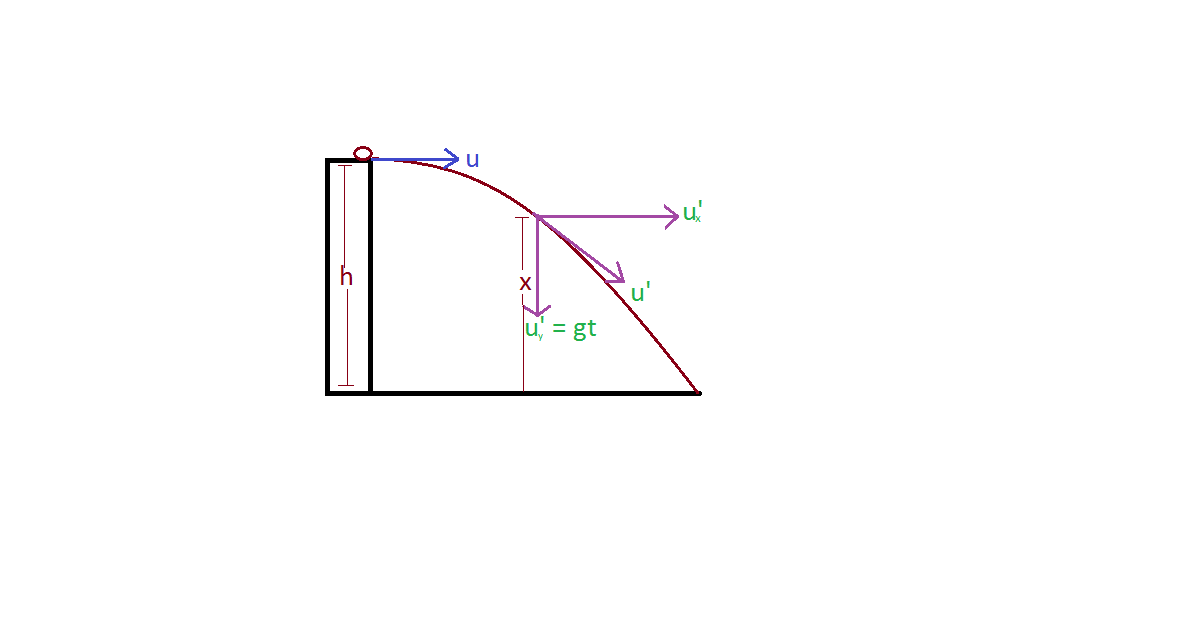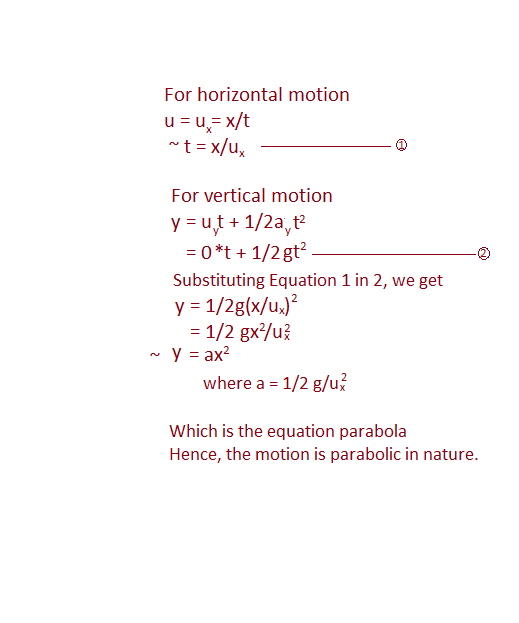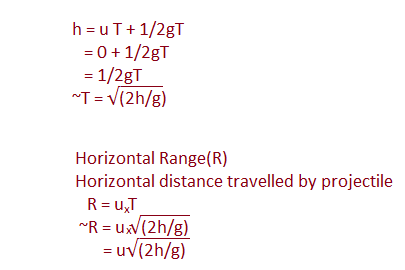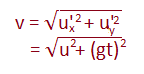Projectile motion and its workings.What is Projectile motion?

It is a two-dimensional motion of an object in the air which is only affected by gravity (no-air resistance) & object launched is called projectile motion.

The path followed by a projectile is called its trajectory. Here, in this motion, we neglect air resistance.

Some terminologies of Projectile motion.

 1 Time of flight(T) The total time taken by the projectile in the air is called the time of flight. It is independent of mass. Time of ascent is always equal to the time of descent in projectile in the same same height. 2 Time of ascent(t) The total time taken by projectile to reach the maximum height is called the time of ascent. (It is only in vertical projectile) 3 Range The total horizontal distance traveled by a projectile is called its range. It is independent of the path followed. It depends upon the time of flight and the velocity thrown.

Types of projectile

1. Horizontal projectile: An object is said to be in horizontal projectile if an object is projected horizontally from a certain height.2. The projectile follows the path of the parabola.• Time of flight(T)• Instantaneous velocity